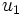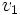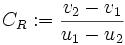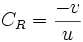# Difference between revisions of "Coefficient of restitution"

## Definition

QUICK PHRASES: bounciness of a collision, final velocity difference divided by initial velocity difference

### For a collision in one dimension

We first consider the case of a head-on collision, which can be considered a collision in one dimension.

Suppose two objects are moving along a one-dimensional axis, collide, and then again move along the same one-dimensional axis. If their initial velocities are (as signed real numbers)$u_1$ and$u_2$ respectively and their final velocities are$v_1$ and$v_2$ respectively, then the coefficient of restitution (abbreviated COR) or bounciness of the collision is defined as:$C_R := \frac{v_2 - v_1}{u_1 - u_2}$.

The coefficient of restitution is also sometimes denoted as$e$.

Note that the ordering of speeds in the numerator is opposite the ordering in the denominator. This reflects the fact that after the collisions, the direction of relative speed changes, because of a bouncing back effect.

### For a collision with a stationary object

If the collision is between a moving object and a heavy stationary object (which remains stationary even after the collision), then the coefficient of restitution is the ratio, with a negative sign, of the final velocity of the moving object to its initial speed. If the initial speed is$u$ and the final speed is$v$, the coefficient of restitution is given by:$C_R = \frac{-v}{u}$.

### For a collision in three dimensions

For a collision in three dimensions, the velocities are vectors rather than scalars, so the above formula does not make sense. In this case, we interpret$u_1,u_2,v_1,v_2$ as the components of velocities in the normal direction, i.e., the direction perpendicular to the plane of contact/tangent plane at the point of contact.

## Constitutive factors and measurement

The coefficient of restitution is dependent on the specific collision and cannot be inferred precisely from the geometry, velocity, and the kind of material in the colliding bodies. However, knowledge of the type of material and the geometry of the bodies usually allows for an approximate estimation of the coefficient of restitution.